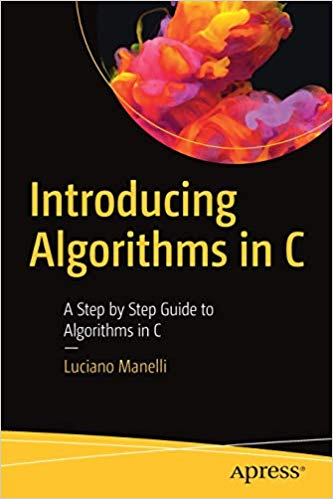# Introducing Algorithms in C – pdf – 电子书免费下载Study elementary and complex algorithms with clear examples and implementations in C. This book introduces data types (simple and structured) and algorithms with graphical and textual explanations. In the next sections, you’ll cover simple and complex standard algorithms with their flowcharts: everything is integrated with explanations and tables to give a step-by-step evolution of the algorithms.

The main algorithms are: the sum of three or n numbers in a loop, decimal-to-binary conversion, maximum and minimum search, linear/sequential search, binary search, bubble sort, selection sort, merging of two sorted arrays, reading characters from a file, stack management, and factorial and Fibonacci sequences.

The last section of Introducing Algorithms in C is devoted to the introduction of the C language and the implementation of the code, which is connected to the studied algorithms. The book is full of screenshots and illustrations showing the meaning of the code.

What You Will Learn

• Implement algorithms in C
• Work with variables, constants, and primitive and structured types
• Use arrays, stacks, queues, graphs, trees, hash tables, records, and files
• Explore the design of algorithms
• Solve searching problems, including binary search, sorting, and bubble/selection sort
• Program recursive algorithms with factorial functions and Fibonacci sequences

Who This Book Is For

Primarily beginners: it can serve as a starting point for anyone who is beginning the study of computer science and information systems for the first time.

ISBN:1484256220

## 锦瑟无端五十弦，一弦一柱思华年

### 觉得文章有用就打赏一下文章作者

#### 支付宝扫一扫打赏#### 微信扫一扫打赏